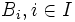# Characteristic subalgebra

View more such properties

## Definition

Suppose$A$ is an algebra (in the universal algebra sense) with a given collection of algebra operations. A subalgebra$B$ of$A$ is termed a characteristic subalgebra if it satisfies the following equivalent conditions:

1. For every automorphism$\sigma$ of$A$,$\sigma(B) \subseteq B$.
2. For every automorphism$\sigma$ of$A$,$\sigma(B)$ is a subalgebra of$B$.
3. For every automorphism$\sigma$ of$A$,$\sigma(B) = B$.

## Particular cases

Variety of interest Characteristic subalgebra in that variety Facts peculiar to characteristic subalgebras in that variety
variety of groups, with the usual universal algebra axiomatization of groups characteristic subgroup characteristic subalgebras are ideals in the variety of groups
variety of Lie rings, with the usual universal algebra axiomatization of Lie rings characteristic Lie subring
variety of powered groups for a set of primes, i.e., fix a set of primes and look at the variety of groups powered over those primes characteristic subgroup that is in addition powered over those primes (note that any powering-invariant characteristic subgroup would satisfy this condition but the converse is not necessarily true, since "powering-invariant" means invariant under powering by all primes the overgroup is powered by, not just the primes for which we are considering the variety.

## Metaproperties

Metaproperty name Satisfied? Proof Statement with symbols
transitive algebra property Yes characteristicity is transitive for any variety of algebras Consider a variety$\mathcal{V}$, an algebra$A$ in$\mathcal{V}$, a characteristic subalgebra$B$ of$A$, and a characteristic subalgebra$C$ of$B$. Then,$C$ is characteristic in$A$.
strongly intersection-closed algebra property Yes characteristicity is strongly intersection-closed for any variety of algebras Consider a variety$\mathcal{V}$, an algebra$A$ in$\mathcal{V}$, and characteristic subalgebras$B_i, i \in I$ of$A$, the intersection$\bigcap_{i \in I} B_i$ is also a characteristic subalgebra of$A$.
strongly join-closed algebra property Yes characteristicity is strongly join-closed for any variety of algebras Consider a variety$\mathcal{V}$, an algebra$A$ in$\mathcal{V}$, and characteristic subalgebras$B_i, i \in I$ of$A$, the join$\langle B_i \rangle_{i \in I}$ is also a characteristic subalgebra of$A$.

## Facts

Note that the notion of subalgebra depends on precisely what operations we consider part of the algebra structure of$A$. For instance, if a group is treated as an algebra in the variety of groups with multiplication, identity, and inverse map operations, the subalgebras are subgroups. If, on the other hand, a group is treated as an algebra in the variety of semigroups (so we just remember the multiplication) then the subalgebras are subsemigroups.

Both the notion of "subalgebra" and the notion of "automorphism" are independent of the choice of subvariety that we are looking at, and hence the notion of "characteristic subalgebra" is also independent. For instance, the notion of characteristic subgroup remains the same whether we are working in the variety of groups or the variety of abelian groups.

## Relation with other properties

### Stronger properties

Property Meaning Proof of implication Proof of strictness (reverse implication failure) Intermediate notions